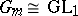# User:Maximilian Janisch/latexlist/Algebraic Groups/Split group

over a field $k$, $k$-split group
A linear algebraic group defined over $k$ and containing a Borel subgroup that is split over $k$. Here a connected solvable linear algebraic group $B$ is called split over $k$ if it is defined over $k$ and has a composition series (cf. Composition sequence) $B = B _ { 0 } \supset B _ { 1 } \supset \ldots \supset B _ { t } = \{ 1 \}$ such that the $B _ { i }$ are connected algebraic subgroups defined over $k$ and each quotient group $B _ { i } / B _ { i + 1 }$ is isomorphic over $k$ to either a one-dimensional torusor to the additive one-dimensional group $G _ { a }$. In particular, an algebraic torus is split over $k$ if and only if it is defined over $k$ and is isomorphic over $k$ to the direct product of copies of the group $G _ { m }$. For connected solvable $k$-split groups the Borel fixed-point theorem holds. A reductive linear algebraic group defined over $k$ is split over $k$ if and only if it has a maximal torus split over $k$, that is, if its $k$-rank coincides with its rank (see Rank of an algebraic group; Reductive group). The image of a $k$-split group under any rational homomorphism defined over $k$ is a $k$-split group. Every linear algebraic group $k$ defined over a field $k$ is split over an algebraic closure of $k$; if $k$ is also reductive or solvable and connected, then it is split over some finite extension of $k$. If $k$ is a perfect field, then a connected solvable linear algebraic group defined over $k$ is split over $k$ if and only if it can be reduced to triangular form over $k$. If $k = 0$, then a linear algebraic group defined over $k$ is split over $k$ if and only if its Lie algebra $L$ is a split (or decomposable) Lie algebra over $k$; by definition, the latter means that the Lie algebra $L$ has a split Cartan subalgebra, that is, a Cartan subalgebra $H \subset L$ for which all eigenvalues of every operator $_ { L } h$, $h \in H$, belong to $k$.
If $G _ { R }$ is the real Lie group of real points of a semi-simple $R$-split algebraic group $k$ and if $G _ { C }$ is the complexification of the Lie group $G _ { R }$, then $G _ { R }$ is called a normal real form of the complex Lie group $G _ { C }$.
There exist quasi-split groups (cf. Quasi-split group) over a field $k$ that are not split groups over $k$; the group $SO ( 3,1 )$ is an example for $k = R$.